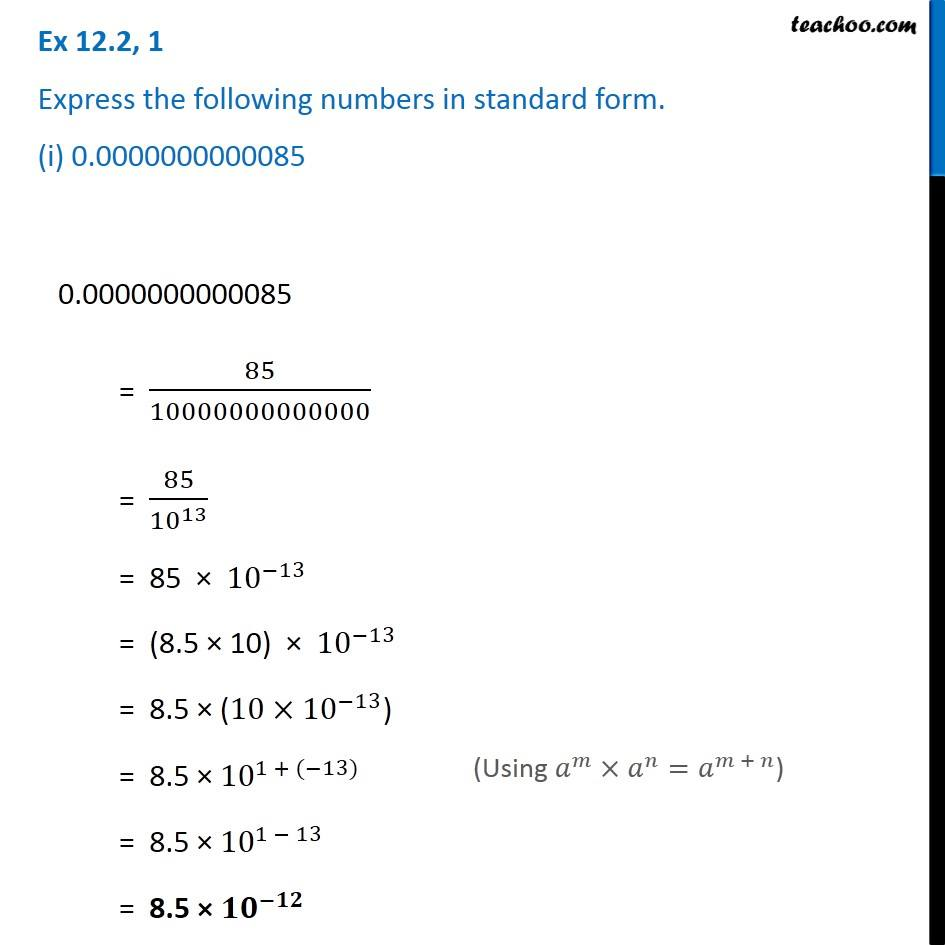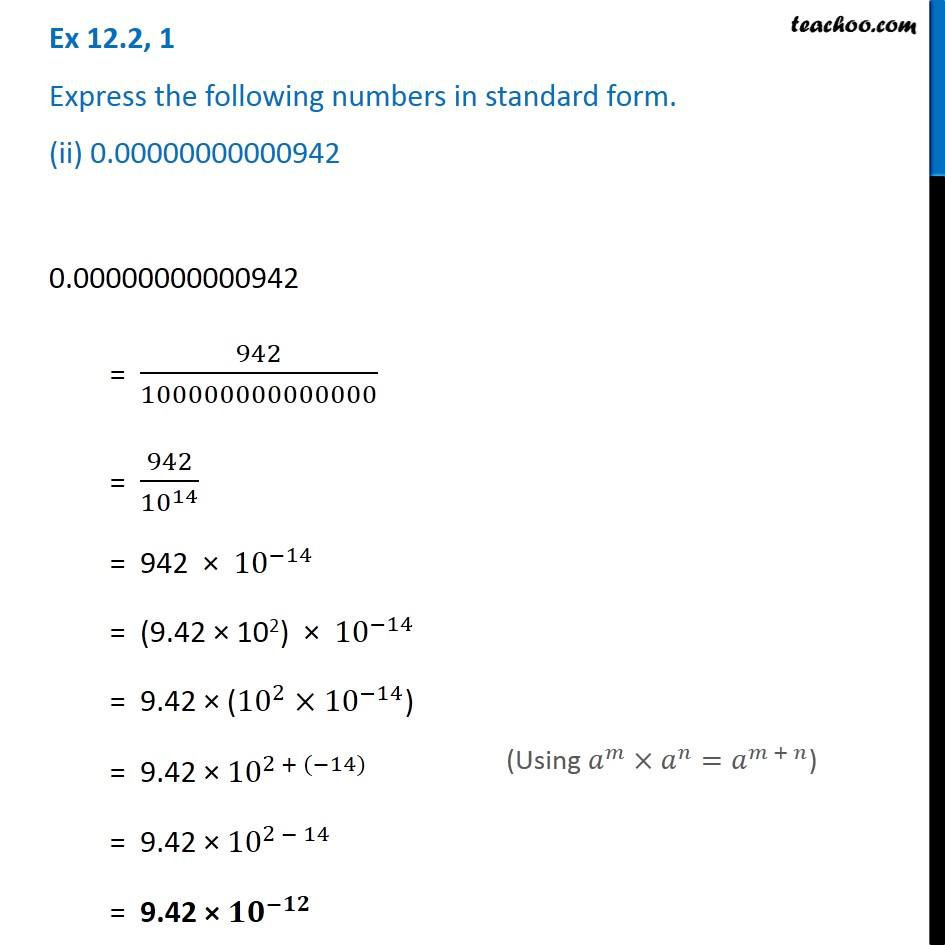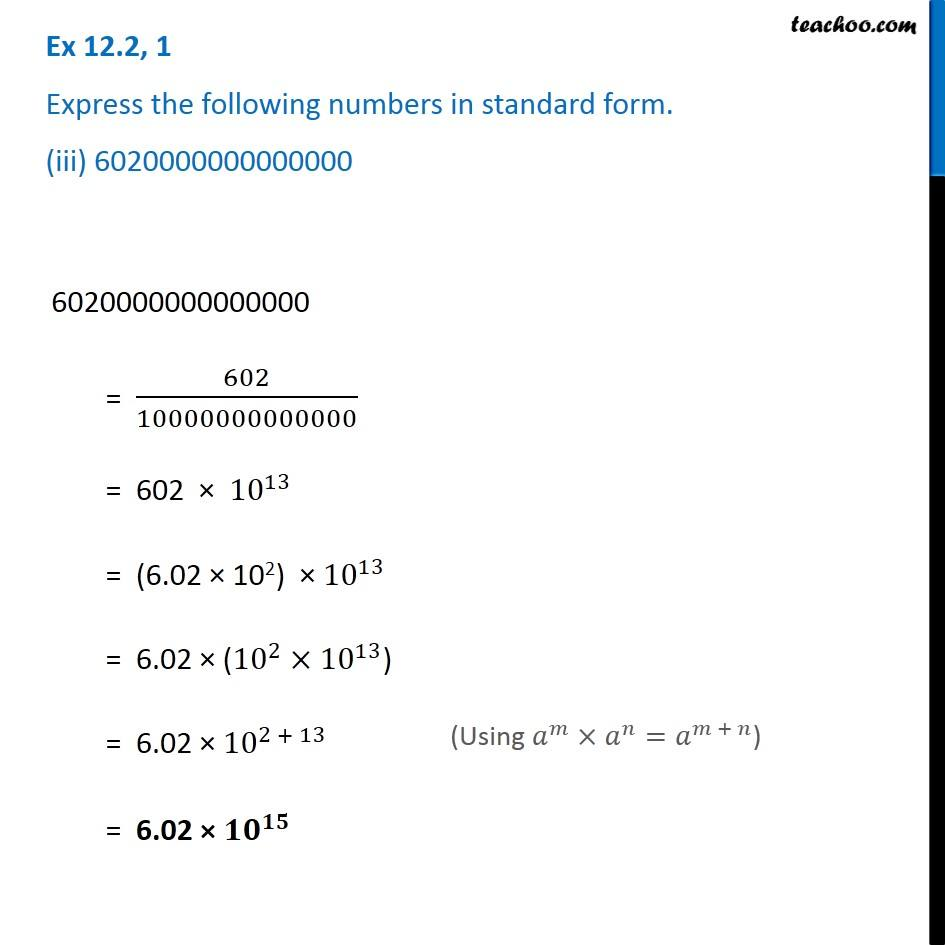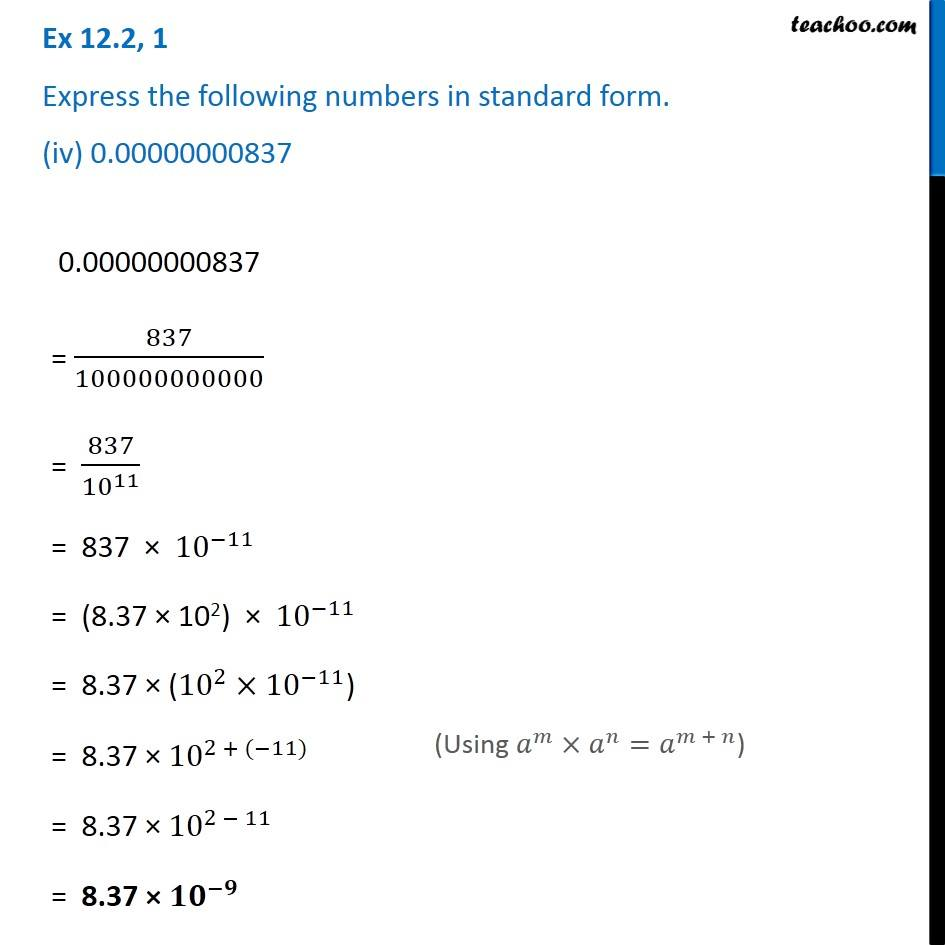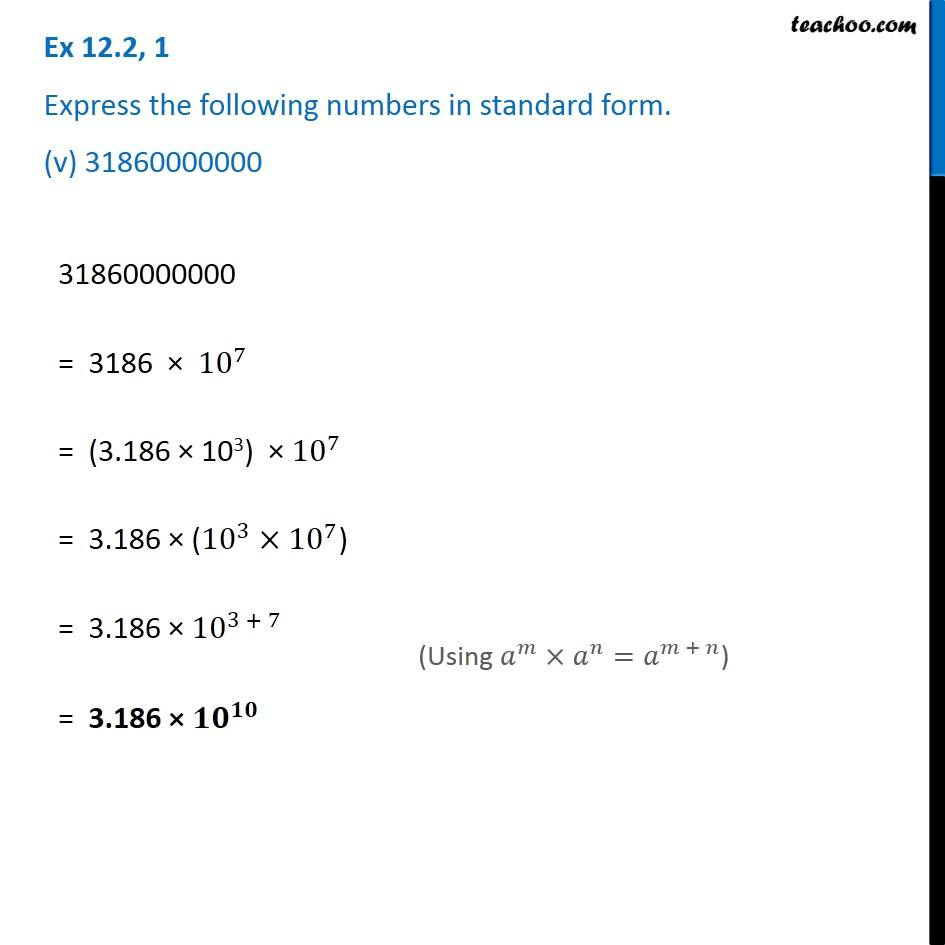1. Chapter 12 Class 8 Exponents and Powers
2. Serial order wise
3. Ex 12.2

Transcript

Ex 12.2, 1 Express the following numbers in standard form. (i) 0.0000000000085 0.0000000000085 = 85/10000000000000 = 85/〖10〗^13 = 85 × 〖10〗^(−13) = (8.5 × 10) × 〖10〗^(−13) = 8.5 × (10×〖10〗^(−13)) = 8.5 × 〖10〗^(1 + (−13)) = 8.5 × 〖10〗^(1 − 13) = 8.5 × 〖𝟏𝟎〗^(−𝟏𝟐) (Using 𝑎^𝑚×𝑎^𝑛=𝑎^(𝑚 + 𝑛)) Ex 12.2, 1 Express the following numbers in standard form. (ii) 0.00000000000942 0.00000000000942 = 942/100000000000000 = 942/〖10〗^14 = 942 × 〖10〗^(−14) = (9.42 × 102) × 〖10〗^(−14) = 9.42 × (〖10〗^2×〖10〗^(−14)) = 9.42 × 〖10〗^(2 + (−14)) = 9.42 × 〖10〗^(2 − 14) = 9.42 × 〖𝟏𝟎〗^(−𝟏𝟐) (Using 𝑎^𝑚×𝑎^𝑛=𝑎^(𝑚 + 𝑛)) Ex 12.2, 1 Express the following numbers in standard form. (iii) 6020000000000000 6020000000000000 = 602/10000000000000 = 602 × 〖10〗^13 = (6.02 × 102) × 〖10〗^13 = 6.02 × (〖10〗^2×〖10〗^13) = 6.02 × 〖10〗^(2 + 13) = 6.02 × 〖𝟏𝟎〗^𝟏𝟓 (Using 𝑎^𝑚×𝑎^𝑛=𝑎^(𝑚 + 𝑛)) Ex 12.2, 1 Express the following numbers in standard form. (iv) 0.00000000837 0.00000000837 = 837/100000000000 = 837/〖10〗^11 = 837 × 〖10〗^(−11) = (8.37 × 102) × 〖10〗^(−11) = 8.37 × (〖10〗^2×〖10〗^(−11)) = 8.37 × 〖10〗^(2 + (−11)) = 8.37 × 〖10〗^(2 − 11) = 8.37 × 〖𝟏𝟎〗^(−𝟗) (Using 𝑎^𝑚×𝑎^𝑛=𝑎^(𝑚 + 𝑛)) Ex 12.2, 1 Express the following numbers in standard form. (v) 31860000000 31860000000 = 3186 × 〖10〗^7 = (3.186 × 103) × 〖10〗^7 = 3.186 × (〖10〗^3×〖10〗^7) = 3.186 × 〖10〗^(3 + 7) = 3.186 × 〖𝟏𝟎〗^𝟏𝟎 (Using 𝑎^𝑚×𝑎^𝑛=𝑎^(𝑚 + 𝑛))

Ex 12.2